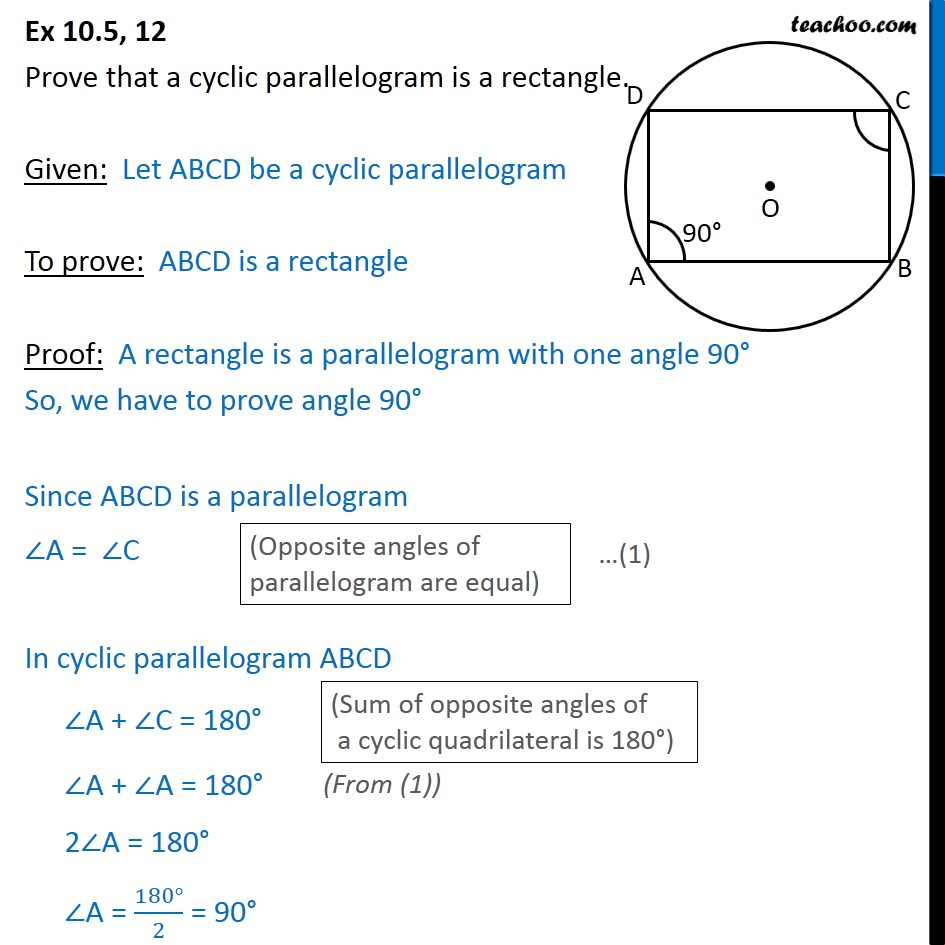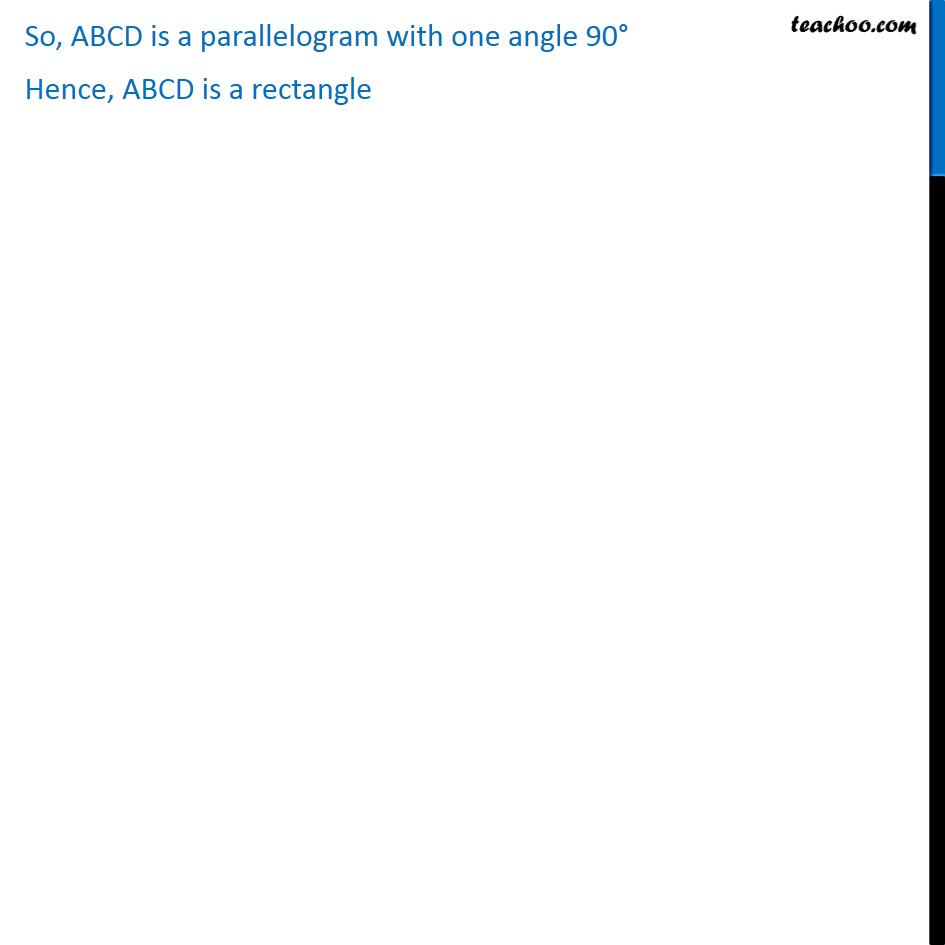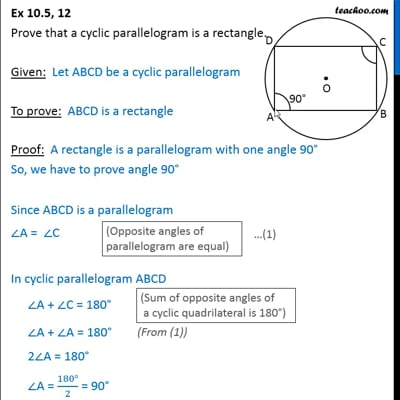Ex 10.5

Chapter 10 Class 9 Circles (Term 2)
Serial order wiseThis video is only available for Teachoo black users

### Transcript

Ex 10.5, 12 Prove that a cyclic parallelogram is a rectangle. Given: Let ABCD be a cyclic parallelogram To prove: ABCD is a rectangle Proof: A rectangle is a parallelogram with one angle 90 So, we have to prove angle 90 Since ABCD is a parallelogram A = C In cyclic parallelogram ABCD A + C = 180 A + A = 180 2 A = 180 A = (180 )/2 = 90 So, ABCD is a parallelogram with one angle 90 Hence, ABCD is a rectangle